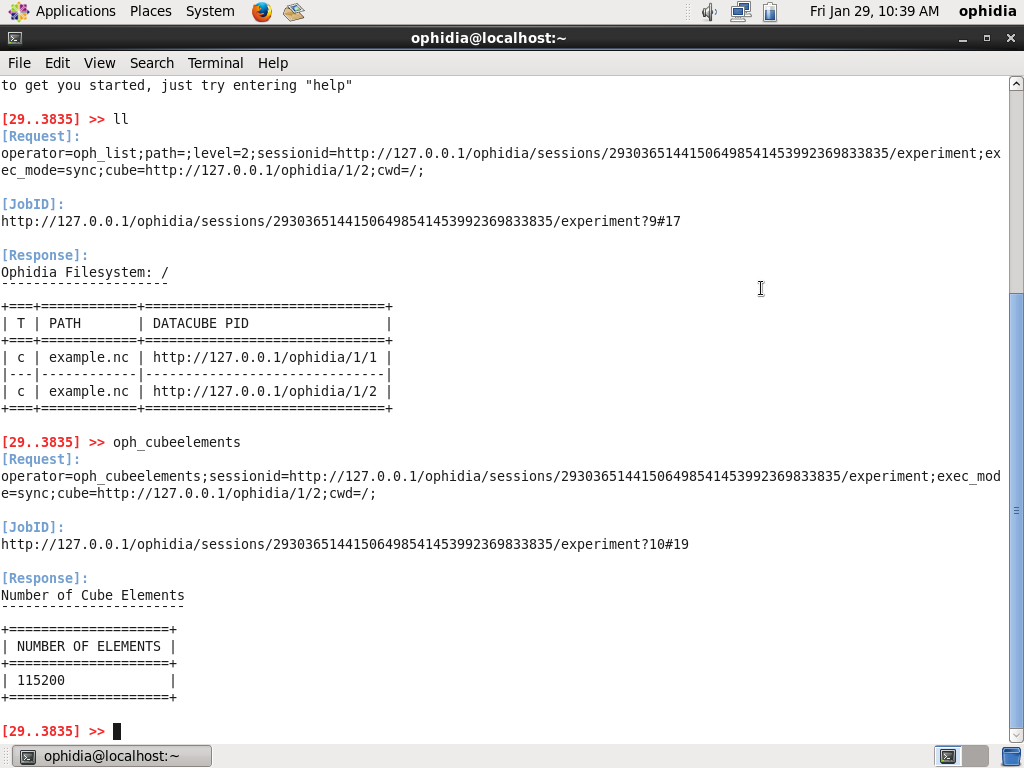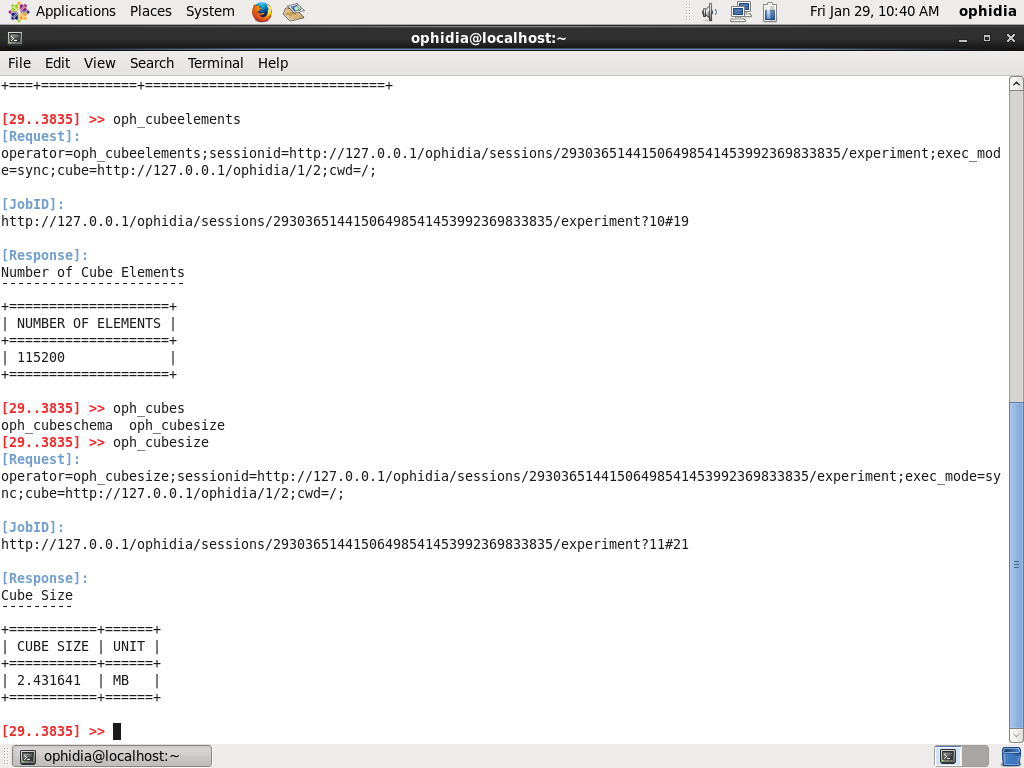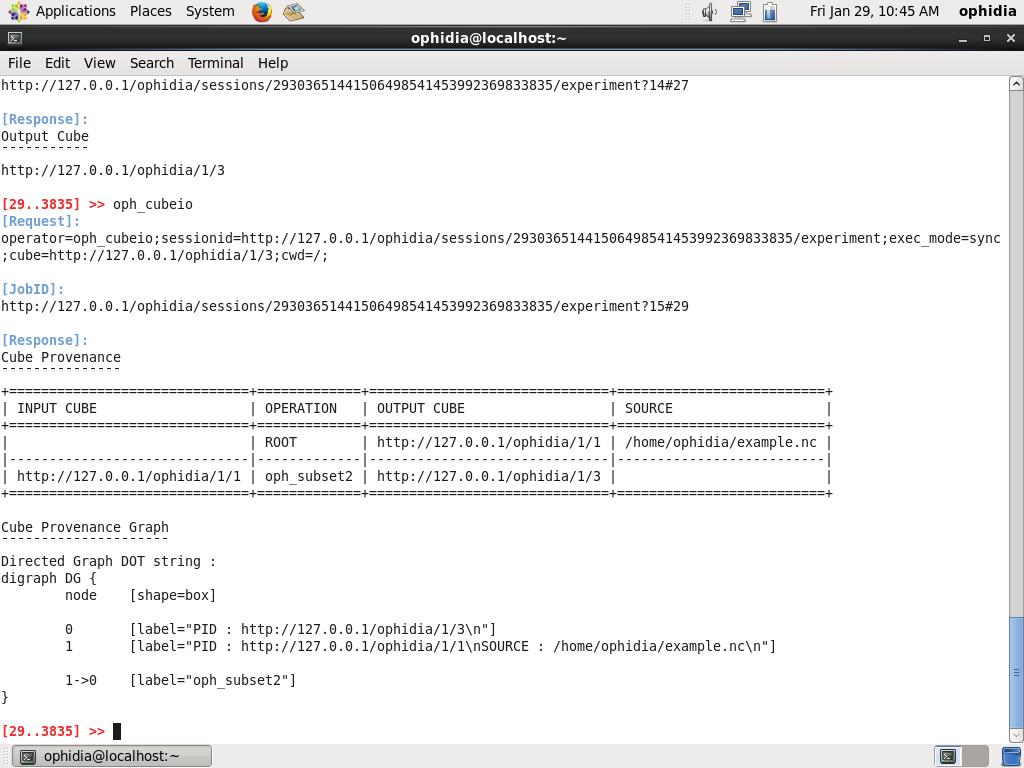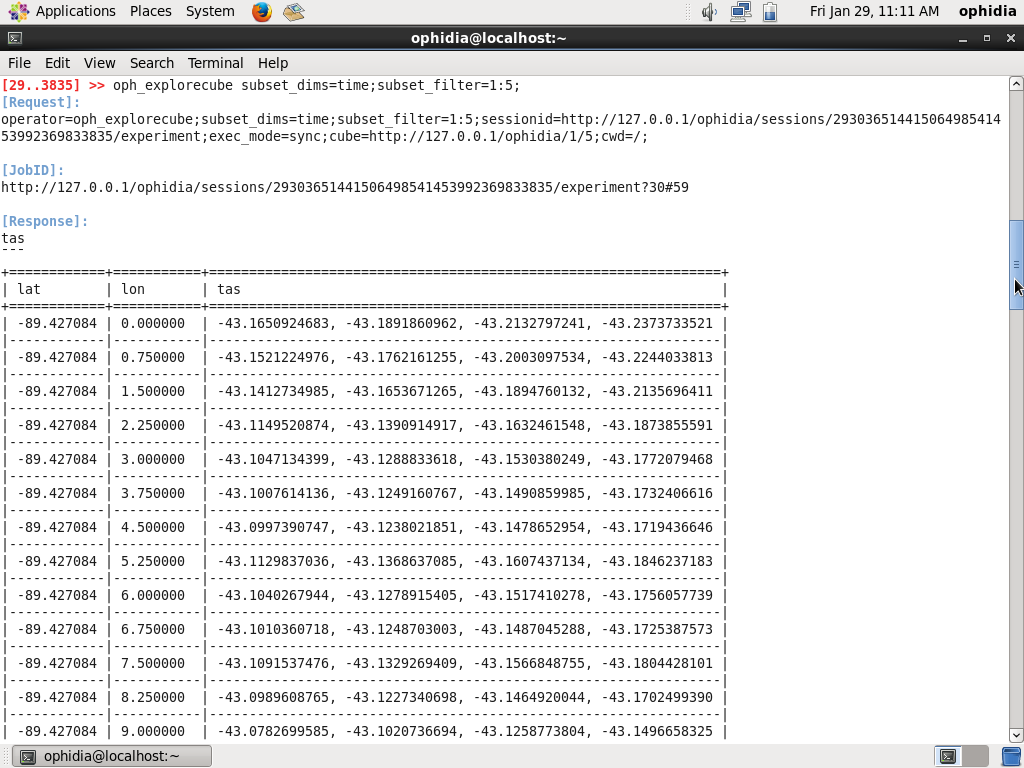# Examples¶

Starting from the Ophidia VMI deployment (see Quick Start section), this section provides a simple Ophidia user session using the Ophidia terminal.

```ll
```

to list the data cubes and containers in your session. Let’s consider PID_1 the identifier of your source data cube (for example http://127.0.0.1/ophidia/1/1).

```oph_reduce2 dim=time;operation=avg;ncores=4;cube=<PID_1>
```

to reduce the whole data cube in a single value for grid point using the average along the time.

```oph_explorecube
```

to visualize the first 100 values.

By default the Ophidia terminal will use the last output data cube PID.

So, apply:

```oph_cubeelements
```

to show the number of measured values```oph_cubesize
```

to show the size of your data cube```oph_cubeschema
```

to show information about the data cube, the dimensions related, the imported variable and types```oph_subset cube=<PID_1>;subset_dims=lat|lon;subset_filter=27:81|-31:39;subset_type=coord;ncores=4;
```

to extract all the values related to Europe only from your source datacube

```oph_cubeio
```

to show the data cube provenance```oph_exportnc2 output_path=<folder_path>;output_name=<filename>;ncores=4;
```

to export the data cube as NetCDF file; you can use “ncdump -h <folder_path>/<filename>.nc” to show the header

```oph_apply cube=<PID_1>;query=oph_sum_scalar('OPH_<variable_type>','OPH_<variable_type>',measure,-273.15);ncores=4;
```

to convert from Kelvin to Celsius degrees.

OPH_<variable_type> represents the type of your data cube variable (e.g. OPH_FLOAT or OPH_DOUBLE). It is listed in the result of the oph_cubeschema operator

```oph_explorecube subset_dims=time;subset_filter=1:5;
```

to show the results for the first 100 grid points and the first 5 time steps```oph_apply cube=<PID_1>;query=oph_gsl_boxplot('OPH_<variable_type>','OPH_<variable_type>',measure);ncores=4;
```

to extract the boxplot from the source data cube

```oph_explorecube
```

to show the boxplot values for the first 100 grid points.